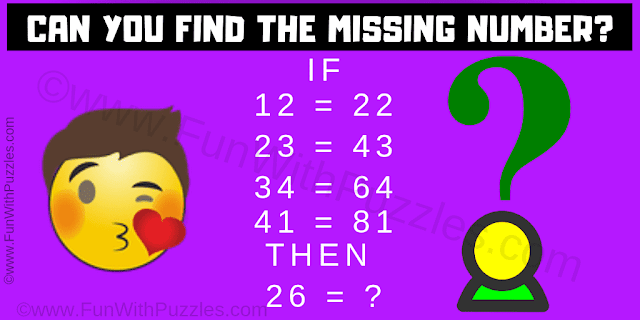This Number Logic Puzzle for Genius Brains who like to solve logical challenges. In this number logic puzzle, there are some number equations. Try to decode the logical reasoning used in these number equations and then find the value of the missing number which will replace the question mark in the last equation.Can you find the missing number?
The answer to this "Puzzle only for Geniuses to Challenge your Brain", can be viewed by clicking on the answer button.